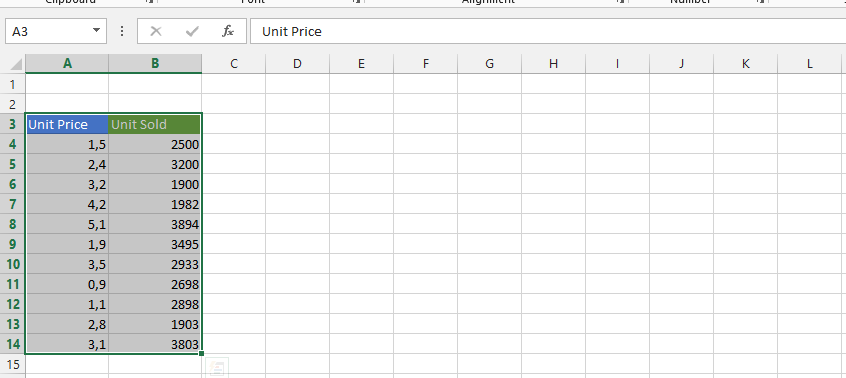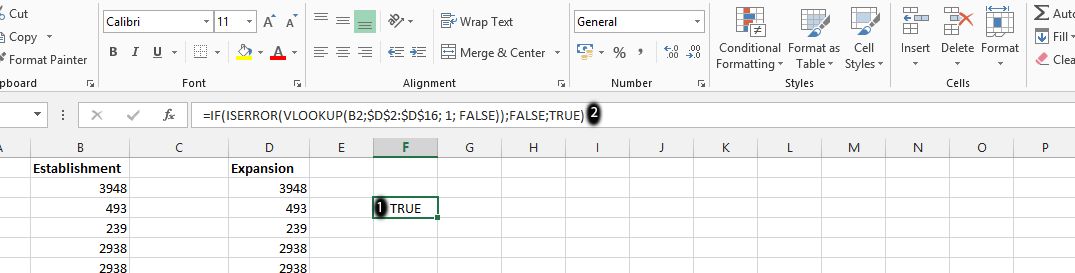#### How to Use Vlookup to Check if Value Exists

The vlookup function can easily help you determine if a value that is in one column is in another.

## Data preparation

This can be useful if the list is very long.

You should have data in one column and have a duplicate in another.Note: You can choose to have another kind of data in the columns.

## Vlookup formula

Click on an empty column (1), and type in =IF(ISERROR(VLOOKUP(B2,\$D\$2:\$D\$16,1,False)),False,True) (2), and then click Enter on the keyboard.Note: This is to know if the value in B2 exists in another column.

## Using the VLOOKUP Function to Find Multiple Values

In addition to checking if a single value exists in another column, the VLOOKUP function can also be used to find multiple values. This can be done by using the wildcard character (*) in the value argument of the VLOOKUP formula.

The wildcard character () can be used to represent any number of characters. For example, the following formula would check if any of the values in the range A2:A10 match the value “123”:

`=VLOOKUP("*123*", A2:A10, 2, False)`

This formula would return the value in the corresponding cell in the range B2:B10 if any of the values in the range A2:A10 match the value “123*.”

The VLOOKUP function can also be used to find multiple values by using the MATCH function. The MATCH function is used to find the position of a value in a range.

The following formula would find the position of the first occurrence of the value “12345” in the range A2:A10:

`=MATCH("12345", A2:A10, 0)`

The MATCH function returns an integer value that represents the position of the value in the range. This value can then be used as the column_index argument of the VLOOKUP function to find the corresponding value in another range.

For example, the following formula would check if the value “12345” exists in the range A2:A10 and return the value in the corresponding cell in the range B2:B10 if the value is found:

`=VLOOKUP(12345, A2:A10, MATCH("12345", A2:A10, 0), False)`

This formula would return the value in the corresponding cell in the range B2:B10 if the value “12345” exists in the range A2:A10.

## How to Check if a Value Exists in Another Sheet

The VLOOKUP function can also be used to check if a value exists in another sheet in Excel. To do this, you will need to use the sheet_name argument in the VLOOKUP formula.

The sheet_name argument specifies the name of the sheet that contains the values that you want to check for. For example, the following formula would check if the value “12345” exists in the range A2:A10 of the sheet named “Sheet2”:

`=VLOOKUP(12345, Sheet2!A2:A10, 2, False)`

The ! symbol is used to separate the sheet name from the range of cells.